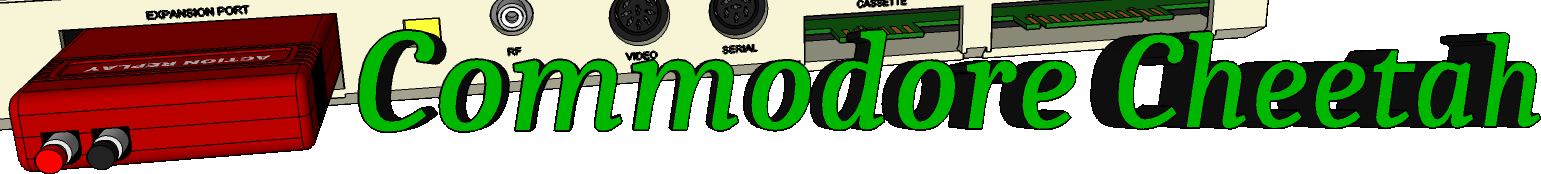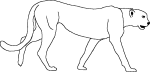Home PageeMail
email5-commodorecheetah.co.uk

Note: You will need to replace the '-' with the @ symbol.
This is to minimize spam.

 My BASIC Quick Reference Guide FOR Abbreviation: F TYPE: Statement FORMAT: FOR = TO [ STEP ] Action: This is a special BASIC statement that lets you easily use a variable as a counter. You must specify certain parameters: the floating-point variable name, its starting value, the limit of the count, and how much to add during each cycle. Here is a simple BASIC program that counts from 1 to 10, PRINTing each number and ENDing when complete, and using no FOR statements: 100 L = 1 110 PRINT L 120 L = 1 + 1 130 IF L <= 10 THEN 110 140 END Using the FOR statement, here is the same program: 100 FOR L = 1 TO 10 110 PRINT L 120 NEXT L 130 END As you can see, the program is shorter and easier to understand using the FOR statement. When the FOR statement is executed, several operations take place. The value is placed in the being used in the counter. In the example above, a I is placed in L. When the NEXT statement is reached, the value is added to the . If a STEP was not included, the is set to + 1. The first time the program above hits line 120, 1 is added to L, so the new value of L is 2. Now the value in the is compared to the . If the has not been reached yet, the program G0es TO the line after the original FOR statement. In this case, the value of 2 in L is less than the limit of 10, so it GOes TO line 110. Eventually, the value of is exceeded by the . At that time, the loop is concluded and the program continues with the line following the NEXT statement. In our example, the value of L reaches 11, which exceeds the limit of 10, and the program goes on with line 130. When the value of is positive, the must exceed the , and when it is negative it must become less than the . NOTE: A loop always executes at least once. EXAMPLES of FOR...TO...STEP...Statement: 100 FOR L = 100 TO 0 STEP -1 100 FOR L = PI TO 6* {pi} STEP .01 100 FOR AA = 3 TO 3

Commodore Cheetah made by Allen Monks, started in the year 2000.